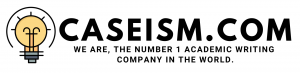# ENCANA CORPORATION: THE COST OF CAPITAL Case Solution & Answer

Common Equity:

Cost of Equity:

The cost of equity is calculated using different approaches. The reason to choose these different approaches is the difference of opinion of both the managers of the EnCana named â€œStevenâ€ & â€œBarbâ€ and due to this reason the cost of equity has been calculated from three different methods, which are Discounted Cash flow (DCF), Capital Asset Pricing Model (CAPM), and from the Bond Yield plus Risk Premium. Neither of these methods supersedes each other and therefore, all three methods are subject to error. The selection of the method depends upon the current strategy of the EnCana.

Using Discounted Cash Flow (DCF:

In this method, the dividends of EnCana Corporation are used. The data provided by the case is from past four years plus the current year, which is deemed to be year 2005. The discounted cash flow approach basically uses dividend that is expected in the subsequent year and discounts it to the current year and that the discount rate is basically the cost of equity of the company, which is in this has to be calculated. The calculation has been provided in the attached excel spreadsheet.

The first step is to calculate the growth rate of the dividends. For this growth rate, Gordon growth model is used. According to this model, the growth rate is calculated from the product of return on equity and retention ratio. The return on equity is calculated from the data given in the exhibit no. 4 of the case. The return on equity is in percentage that shows the return earned by the company on the investment, which is financed by the common shareholders or retained earnings. The next component in the calculation of the growth is the retention ratio, which shows the amount of earnings retained by the company. This is calculated by subtracting the payout ratio from the 100% (considered as 1). In this case, the payout ratio is also taken as an average of 5 years data, which is equal to 8% and after subtracting from 100%, it shows the remaining 92% retention ratio.This growth rate is used in calculating the expected dividend for the next year 2006. The expected dividend is equal to \$0.30 per share. The current market price is 52.56. The formula for this is shown in the given exhibit. The cost of equity in this case is equal to 8.48%. The assumption of using this method is that the company will pay this dividend or will retain its earnings by this amount for the foreseeable future.

Using CAPM:

The capital asset pricing model is the frequent model used from these three models. This is because this model helps the company in determining its changing risk due to the changes in the capital structure. The change in the capital structure is due to the adoption of a new projectthat requires substantial investment, which is financed with the mix of debt and equity as reflected in this case. EnCana is currently seeking to take a project in the near future. The project will be financed by the mix of debt and equity as per the companyâ€™s old financing policy.

The first component of this model is the estimation of beta that shows the companyâ€™s overall risk from the market perspective in order to earn that return. The beta in this case is given, which is equal to 1.27.This beta describes the situation that the company is currently more risky than the overall market. This is why many companies prefer to choose this model as it helps the company to reflect the overall changes in their cost of capital due to the proposed project…………………………

This is just a sample partial work. Please place the order on the website to get your own originally done case solution